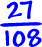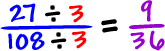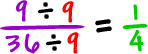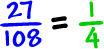Let's try one:

Let's reduceWhat goes into the numerator AND the denominator?

3 goes in... So, let's divide it out!Hey, now it's easy! Do you see that 9 goes in?soThe best way to do it is to get the GCF (greatest common factor) of 27 and 108...

 The factors of 27: 1, 3, 9, 27 The factors of 108: 1, 2, 3, 4, 6, 9, 12, 18, 27, 36, 54, 108

The GCF is 27.

If we divide by the GCF, we can do it in one shot:But, you know what?

If you can't find the GCF, just start dividing out stuff you see like we did at first.